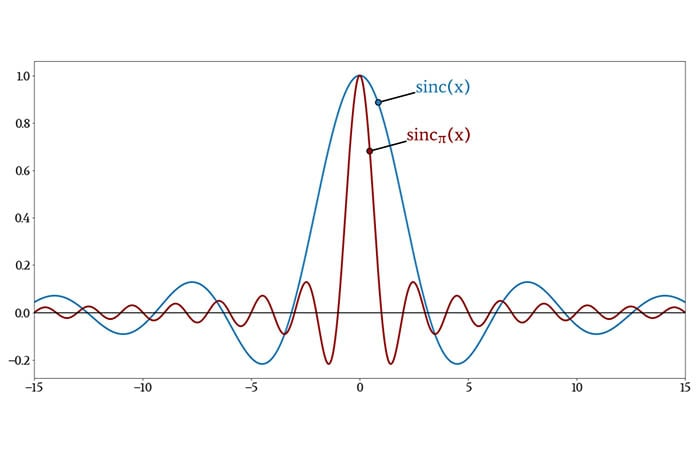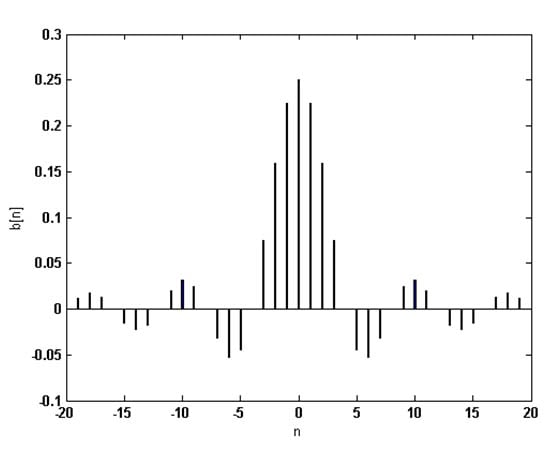Technical Article

# What Is the Sinc Function and Why Is It Important in Electrical Engineering?

September 23, 2020 by Robert Keim

## This Frequent Engineering Question gives a quick overview of a mathematical function that appears frequently in digital signal processing.

### The Sinc Function and Its Normalized Variant

The mathematical formulation of the sinc function, also known as the cardinal sine function, is written as follows:

$\text{sinc}(x)=\frac{\sin(x)}{x}$

The function shown above is undefined for x = 0, and consequently we need to define sinc(0) based on the limit as x approaches 0, which is 1. Thus,

$\text{sinc}(x)=\begin{cases}1 & \text{for } x = 0\\\frac{\sin(x)}{x} & \text{otherwise}\end{cases}$

In the context of digital signal processing, we often use an alternative form in which the independent variable is multiplied by π:

$\text{sinc}_\pi (x)\equiv\text{sinc}(\pi x)=\begin{cases}1 & \text{for } x = 0\\\frac{\sin(\pi x)}{\pi x} & \text{otherwise}\end{cases}$

This second form is called the normalized sinc function, because the definite integral over the entire range of x is equal to 1:

$\int_{-\infty}^{\infty}\text{sinc}_\pi(x)dx=1$

I mentioned that the normalized version is common in digital signal processing. When we’re dealing with discrete data instead of a continuous-time variable, the normalization is expressed as follows:

$\sum_{n=-\infty}^{\infty}\text{sinc}_\pi[n]=1$

The following plot shows the shape of the sinc function, and it also conveys the difference created when we multiply the independent variable by π.### The Sinc Function in Signal Processing

The Fourier transform of the sinc function is a rectangle centered on ω = 0. This gives sinc(x) a special place in the realm of signal processing, because a rectangular shape in the frequency domain is the idealized “brick-wall” filter response. In other words, sinc(x) is the impulse response of an ideal low-pass filter.

The use of the sinc function in filtering applications is more apparent in the digital domain. The following diagram illustrates the similarity between the impulse response of a FIR filter and a plot of sinc(x).The Fourier transform of the sinc function is a rectangle, and the Fourier transform of a rectangular pulse is a sinc function. If we need to shorten a discrete-time signal for the purpose of spectral analysis, we can multiply it by a rectangular window, and this operation is equivalent to convolving the Fourier transform of the signal with a sinc function.

The sinc function also appears in analysis of digital-to-analog conversion. An idealized reconstruction of an analog signal is a sequence of impulses, but real-life DACs produce “staircase” waveforms by applying a zeroth-order hold to the output samples. In the frequency-domain, the zeroth-order hold results in an output spectrum that is equal to the idealized spectrum multiplied by the sinc function.

1 Comment• V
vanderghast September 25, 2020

An oscilloscope offers the possibility to fill the curve missing points by interpolating between the sampled points with sinc instead of displaying straight lines segments. I have always wonder how this is done. Does that involves too much development to explain how it is done and what are the possible disadvantages (and advantages) ?

Like.